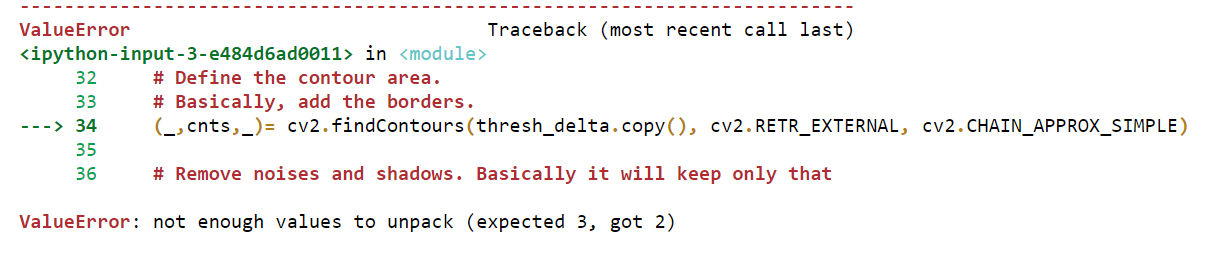System information (version)

• Operating System / Platform => : Windows 10 64 Bit
• Compiler => : Jupyter Notebook (Anaconda)

Detailed description

ValueError: not enough values to unpack (expected 3, got 2)
When trying to run the code below (Motion Detector using Python)

Program

```import cv2
import time

first_frame = None
# Create a VideoCApture object to record video using web Cam
video  = cv2.VideoCapture(0)

while True:

# Convert the frame to gray scale
gray= cv2.cvtColor(frame, cv2.COLOR_BGR2GRAY)

# Convert the gray scale image to GaussianBlur
gray = cv2.GaussianBlur(gray, (21,21),0)

# To store first image/frame of video
if first_frame is None:
first_frame = gray
continue

# Calculate the difference between the first frame and other frames
delta_frame = cv2.absdiff(first_frame, gray)

# Provides a threshold value, such that it will convert
# the difference value with less than 30 to black.
# If the difference is greater than 30 it will convert
# those pixels to white
thresh_delta = cv2.threshold(delta_frame, 30,255, cv2.THRESH_BINARY)
thresh_delta = cv2.dilate(thresh_delta , None , iterations = 0)

# Define the contour area.
(_,cnts,_)= cv2.findContours(thresh_delta.copy(), cv2.RETR_EXTERNAL, cv2.CHAIN_APPROX_SIMPLE)

# Remove noises and shadows. Basically it will keep only that
# part white, which has area greater than 1000 pixels.

for contour in cnts:
if cv2.contourArea(contour) <1000:
continue

# Creates a rectangular box around the object in the frame
(x,y,w,h) = cv2.boundingRect(contour)
cv2.rectangle(frame, (x, y), (x+w, y+h), (0, 255, 0), 3)

cv2.imshow('frame', frame)
cv2.imshow('capturing', gray)
cv2.imshow('delta',delta_frame)
cv2.imshow('thresh', thresh_delta)

key = cv2.waitKey(1)
if key == ord('q'):
break
video.release()
cv2.destoyAllWindows
```

Error Screenshot:Jun 23 2,036 views
Thanks ,it works
I Have the same problem

(new, cnts, _) = cv2.findContours(edged.copy(), cv2.RETR_LIST, cv2.CHAIN_APPROX_SIMPLE)
ValueError: not enough values to unpack (expected 3, got 2)

pls reply what should I do now

## 1 answer to this question.

+1 vote

Make the following changes in your script, it will work:

`(cnts, _) = cv2.findContours(thresh_delta.copy(), cv2.RETR_EXTERNAL, cv2.CHAIN_APPROX_SIMPLE)`answered Jun 24 by
• 68,480 points
Thanks, it works for now.

## Example to run KNN algorithm using python

Have a look at this one: from sklearn.datasets ...READ MORE

## Ignore the NaN and do the linear regression on remaining values

Yes, you can do this using statsmodels: import ...READ MORE

## How do I convert a pandas dataframe to a numpy array using python?

Try something like this: df.values array([[nan, 0.2, nan], ...READ MORE

## ValueError: Input contains NaN, infinity or a value too large for dtype('float32')

Assuming X_test is a pandas dataframe, you ...READ MORE

## how do i change string to a list?

suppose you have a string with a ...READ MORE

+1 vote